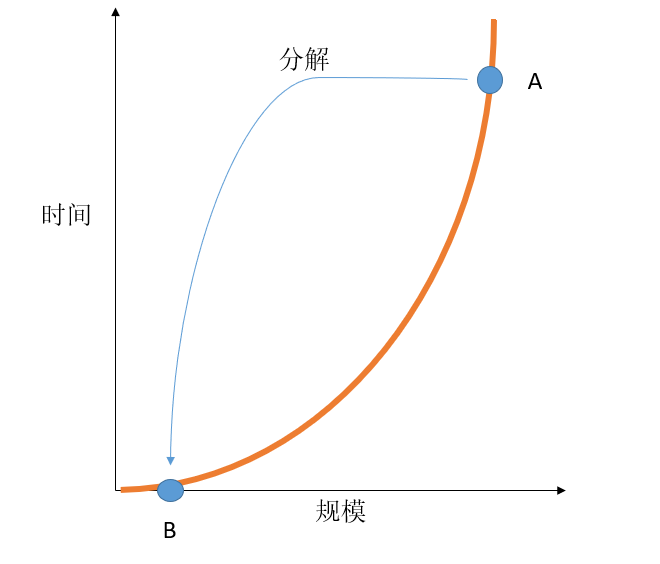# 归并排序、jensen不等式、非线性、深度学习## 归并排序

def merge_sort(arr): “““ input : unsorted array output: sorted array in ascending order “““ if len(arr) <= 1:  return arr half = len(arr)//2 left = merge_sort(arr[0:half]) right = merge_sort(arr[half:]) left_tail ，right_tail = 0 ， 0 sorted_arr = [] while left_tail < len(left) and right_tail < len(right):  if left[left_tail] < right[right_tail]:sorted_arr.append(left[left_tail])left_tail  = 1  else:sorted_arr.append(right[right_tail])right_tail  = 1 if left_tail < len(left):  sorted_arr  = left[left_tail:] else:  sorted_arr  = right[right_tail:] return sorted_arr

## 非线性，Jensen 不等式$f(\sum_{i=1}^{N}\lambda_{i}x_{i}) < \sum_{i=1}^{N}\lambda_{i}f(x_{i})$